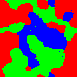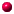# Monte Carlo simulaatiomenetelmät Monte Carlo simulation methodsLecturer: Kari RummukainenLectures: 2h/week, Tue 10-12Exercises: Ahti Leppänen, Thu 10-12 (not every week)Description: The course covers the basics of Monte Carlo simulations, with concrete applications to simple spin models (Ising model, XY model) and other selected models. The emphasis is on practical issues: update algorithms, measurements, error analysis. We will also consider some modern Monte Carlo methods, like reweighting, multicanonical methods, and cluster algorithms. The topics are applicable to almost any area of physics.Requirements: Basics of numerical methods and statistical physics. Knowledge of some programming language (Fortran, C, C++, Java ...) is needed for the exercises.Exercises: The exercises consist of mathematical/theoretical problems and programming tasks.Problems? Come to talk to K.R. at TE317 at any time. A.L. can be reached at "Tuutortupa" as follows: Mon 12 - 13, Thu 13 - 14, Fri 10 - 11.

HomeworkHomework 1, to be returned by 27.9. Example results and code(C++) can be found from here.Homework 2, to be returned by 18.10. Example results and code(C++) can be found from here. (Updated 1.11)Homework 3, to be returned by 1.11. Example results and code(C++) can be found from here. (Updated 29.11)Homework 4, to be returned by 29.11. NOTE: slightly corrected version 26.11! Example results and code(C++) can be found from here.

Lecture notesPart 1: Monte Carlo integration and random numbers (note added) (2.10: Corrected small error in Schrage's formula, p. 27. Thanks to D. Fernandez)Part 2: Monte Carlo simulation (note added to older version in 23.10: addition to sect. 4.15, Autocorrelations. Included in the present version of part 2)Part 3: Monte Carlo of particle systemsPart 4: ReweightingPart 5: Jackknife and bootstrap; finite size scalingPart 6: Multicanonical methods and cluster algorithms (NOTE: modifications also in the multicanonical part 26.11.)Some example programs are available hereFun with the Ising model: X-windows Ising model demonstration program (requires mersenne.h and mersenne_inline.c from the link above).Textbooks and other course material:
There is no single textbook which covers the course material. Lecture notes will be the primary material. Additional material:

• W. H. Press, S. A. Teukolsky, W. T. Vetterling, and B. P. Flannery, Numerical Recipes in C/Fortran; The Art of Scientific Computing, Cambridge University Press, New York, second edition, 1995
An excellent swiss-army-knife of a book for numerical analysis.
Online version of Numerical Recipes
• Gould, Tobochnik: An Introduction to Computer Simulation Methods: Applications to Physical Systems, Harvey Gould, et al.
relatively simple introduction to computer simulation methods, including Monte Carlo. Uses a dialect of BASIC(!)
• K. Binder und D.W. Heermann, Monte Carlo Simulation in Statistical Physics, Springer Series in Solid-State Sciences 80, Springer 1988. A bit more advanced and mathematical textbook.
• M. Creutz: Quarks, Gluons and Lattices, Cambridge Univ. Press 1983. Introduction to field theory on the lattice, not much about simulation methods.
• Other very useful lecture notes available on the web:
Laskennallinen fysiikka (761668S), Fysiikan syventävä opintojakso Oulun yliopistossa. Sisältää paljon samoja aiheita kuin nämä luennot. (In Finnish)
Excellent lecture notes by Kai Nordlund, University of Helsinki. Check it!
• Another set of lecture notes: Monte Carlo Simulation for Statistical Physics by Paul Coddington, Syracuse University.